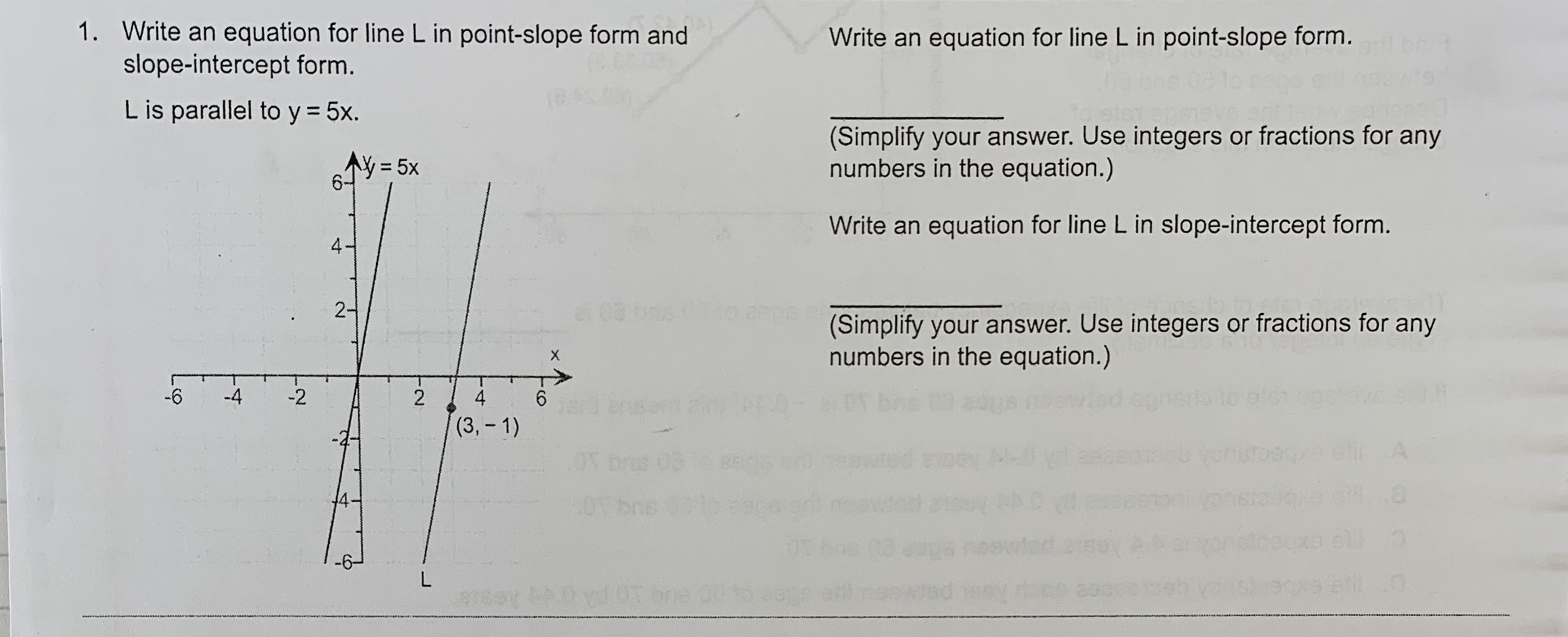# 1. Write an equation for line L in point-slope form andslope-intercept form.Write an equation for line L in point-slope form.L is parallel to y 5x.(Simplify your answer. Use integers or fractions for anynumbers in the equation.)AY=5xWrite an equation for line L in slope-intercept form.42ei 08o a0p(Simplify your answer. Use integers or fractions for anynumbers in the equation.)X-4-6-224et an br(3,-1)AngeyL0T bos86T4bos

Question
17 views

Can someone help me with this problem?help_outlineImage Transcriptionclose1. Write an equation for line L in point-slope form and slope-intercept form. Write an equation for line L in point-slope form. L is parallel to y 5x. (Simplify your answer. Use integers or fractions for any numbers in the equation.) AY=5x Write an equation for line L in slope-intercept form. 4 2 ei 08 o a0p (Simplify your answer. Use integers or fractions for any numbers in the equation.) X -4 -6 -2 2 4 et an br (3,-1) A ngeyL 0T bos 86T 4 bos fullscreen
check_circle

Step 1

Parallel lines have same slope.

So we find slope of y=5x by comparing with y=mx+b

m=5, b=0

So, slope=m=5

Step 2

The L  line has slope=5 and passes through (3,-1)

We use the point slope f...

### Want to see the full answer?

See Solution

#### Want to see this answer and more?

Solutions are written by subject experts who are available 24/7. Questions are typically answered within 1 hour.*

See Solution
*Response times may vary by subject and question.
Tagged in

### Other